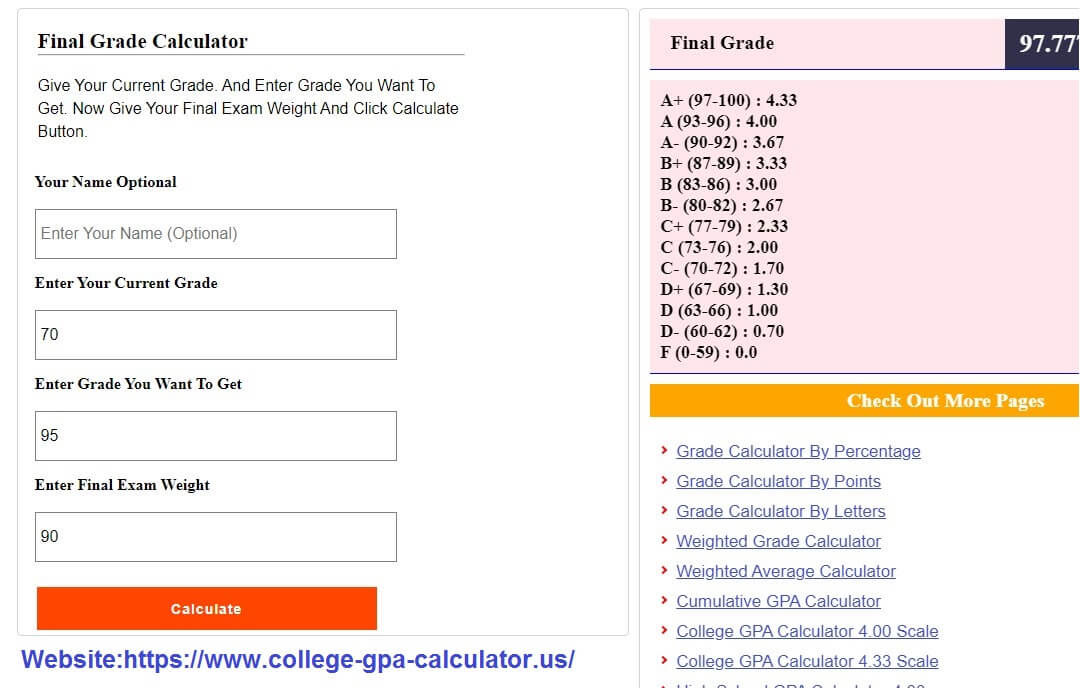GPA and CGPA Calculators

#### Check Out More Pages

It Simple The final exam grade Should Be equal to the All required grade, minimize 100%. And minimize the final exam weight (w) times the current grade (g), Now divided by the final exam weight (w): That's It.

### How To Calculate Final Grade